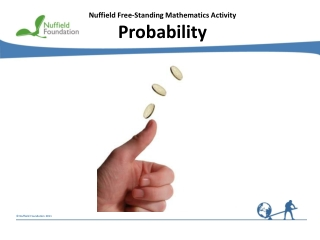DownloadDownload PresentationNuffield Free-Standing Mathematics Activity Probability

# Nuffield Free-Standing Mathematics Activity Probability

Download Presentation## Nuffield Free-Standing Mathematics Activity Probability

- - - - - - - - - - - - - - - - - - - - - - - - - - - E N D - - - - - - - - - - - - - - - - - - - - - - - - - - -
##### Presentation Transcript

1. Nuffield Free-Standing Mathematics Activity Probability

2. Probability What is the probability that the bus does not come on time? What is the probability of all the coins landing heads up?’ Sometimes you can work out probabilities, but sometimes you need to use an experiment or past experience. In this activity you will use both methods.

3. Probability How can you estimate: • the probability that a drawing pin lands point up if you drop it onto the floor? • the probability of a train being late? • the probability of having an accident at home? Experimental probability If drawing pin lands point up in 140 out of 200 throws = 0.7 Think about ... In what other situations can data help you to estimate probabilities? The more data you have, the better the estimate.

4. must be equally likely 0 1 P(even) P(less than 7) P(6) P(7) P(more than 2) Theoretical probability If a fair dice is thrown P(6) Think about ...How do these use the fact that the dice is fair? P(7) = 0 P(even) P(less than 7) = 1 P(more than 2) Probability line (impossible) (certain)

5. P(E) = 1 – P(E) P(E doesn’t happen) Think about... What is the probability that the drawing pin lands point down? Expectation Expected number of trials in which E occurs = P(E)  total number of trials In 300 throws of a fair dice = 50 expected number of sixes = 150 expected number of even scores = 200 expected number of scores above 2

6. Possible outcomes – tossing two coins 2nd coin H T HH HT H 1st coin T TH TT H H T H T T 1st coin 2nd coin List possibilities Use a table HH, HT, TH, TT HH TH TT Use a tree diagram HH Probabilities if coins are fair P(two Hs) HT TH P(H&T in any order) TT P(at least one T)

7. Reflect on your work Explain how relative frequency is used to estimate probabilities. Give some examples. Write down a formula for the theoretical probability of an event E. Why is it important to assume that dice and coins are fair when you are calculating theoretical probabilities? Describe three ways of finding probabilities of combined events.